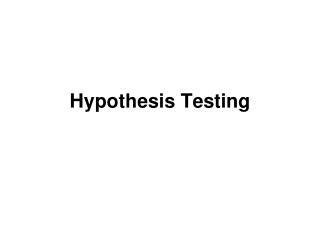DownloadDownload PresentationHypothesis Testing

# Hypothesis Testing

Download Presentation## Hypothesis Testing

- - - - - - - - - - - - - - - - - - - - - - - - - - - E N D - - - - - - - - - - - - - - - - - - - - - - - - - - -
##### Presentation Transcript

1. Hypothesis Testing

2. Central Limit Theorem Hypotheses and statistics are dependent upon this theorem

3. Central Limit Theorem To understand the Central Limit Theorem we must understand the difference between three types of distributions…..

4. Of particular interest is the “normal distribution”

5. Different populations will create differing frequency distributions, even for the same variable…

6. There are three fundamental types of distributions: • Population distributions

7. There are three types of distributions: • Population distributions

8. There are three types of distributions: • Population distributions

9. There are three types of distributions: • Population distributions

10. There are three types of distributions: • Population distributions

11. There are three types of distributions: • Population distributions • Sample distributions

12. There are three types of distributions: • Population distributions • Sample distributions

13. There are three types of distributions: • Population distributions • Sample distributions • Samplingdistributions

14. Population distributions The frequency distributions of a population.

15. 2. Sample distributions The frequency distributions of samples. The sample distribution should look like the population distribution….. Why?

16. 2. Sample distributions The frequency distributions of samples.

17. 3. Samplingdistributions The frequency distributions of statistics.

18. 2. Sample distributions The frequency distributions of samples. The sampling distribution should NOT look like the population distribution….. Why?

19. Suppose we had population distributions that looked like these:

20. Say the mean was equal to 40, if we took a random sample from this population of a certain size n… over and over again and calculated the mean each time……

21. We could make a distribution of nothing but those means. This would be a sampling distribution of means.

23. 1. What would be the mean of all those means?

24. 2. If the population mean was 40, how many of the sample means would be larger than 40, and how many would be less than 40?

25. Regardless of the shape of the distribution below, the sampling distribution would be symmetrical around the population mean of 40.

26. 3. What will be the variance of the sampling distribution?

27. The means of all the samples will be closer together (have less variance) if the variance of the population is smaller.

28. The means of all the samples will be closer together (have less variance) if the size of each sample (n) gets larger.

29. n = number of samples Sample

30. So the sampling distribution will have a mean equal to the population mean, and a variance inversely proportional to the size of the sample (n), and proportional to the variance of the population. http://www.khanacademy.org/math/statistics/v/central-limit-theorem http://www.khanacademy.org/math/statistics/v/sampling-distribution-of-the-sample-mean

31. Central Limit Theorem

32. Central Limit Theorem If samples are large, then the sampling distribution created by those samples will have a meanequal to the population mean and a standard deviation equal to the standard error.

33. Sampling Error = Standard Error

34. This makes inferential statistics possible because all the characteristics of a normal curve are known.

35. http://www.statisticalengineering.com/central_limit_theorem.htmhttp://www.statisticalengineering.com/central_limit_theorem.htm A great example of the theorem in action….

37. Hypothesis Testing: A statistic tests a hypothesis: H0

38. Hypothesis Testing: A statistic tests a hypothesis: H0 The alternative or default hypothesis is: HA

39. Hypothesis Testing: A statistic tests a hypothesis: H0 The alternative or default hypothesis is: HA A probability is established to test the “null” hypothesis.

40. Hypothesis Testing: 95% confidence: would mean that there would need to be 5% or less probability of getting the null hypothesis; the null hypothesis would then be dropped in favor of the “alternative” hypothesis.

41. Hypothesis Testing: 95% confidence: would mean that there would need to be 5% or less probability of getting the null hypothesis; the null hypothesis would then be dropped in favor of the “alternative” hypothesis. 1 - confidence level (.95) = alpha

42. Alpha

43. Errors:

44. Errors: Type I Error: saying nothing is happening when something is: p = alpha